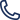530-820-3390 530-820-3390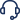Support

## Math function

This function is used to perform arithmetic operations (+,-,*,/) between given two numbers. Use sum for addition, minus for subtraction, multiply for multiplication and divide for division in the math function.

Syntax: \${__math(operator,first number,second number)}

Example: \${__math(sum,5,10)} \${__math(minus,5,3)} \${__math(multiply,5,3)} \${__math(divide,10,2)}

Two numbers used in this function can be static or dynamic (coming from variables etc.)

Edit parameter section and click on Form, select functions from the dropdown and select math function. Select the arithmetic operation you want to perform and enter the operands as shown below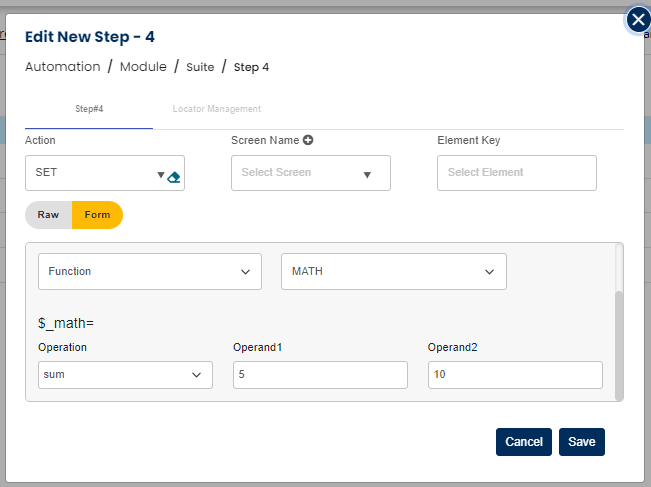After completing this step, step will look as below: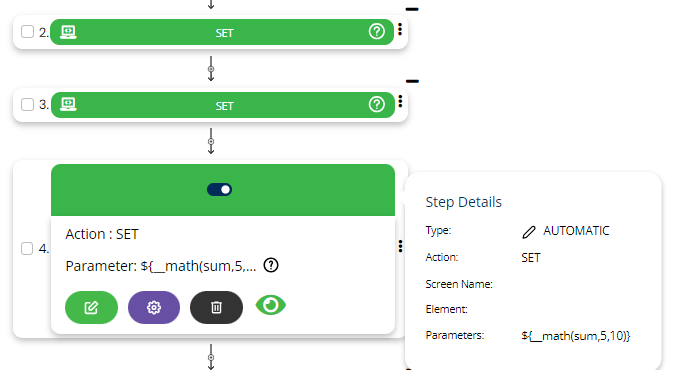Example: \${__math(multiply,\${x},\${y})} Here, x and y are two local variables.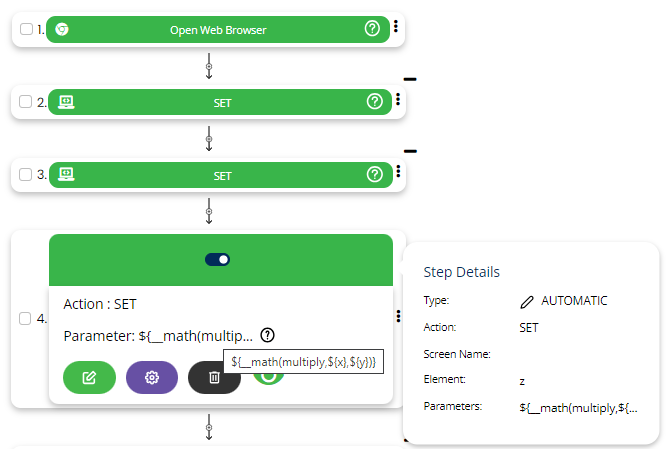In the above example two variables are set x and y whose sum will be calculated and output will be stored in variable z defined in step four.

Once variable z is assigned with the result you can use it in your further steps as required.